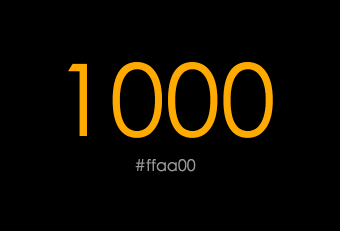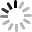# SVG D3.js - transition ( tween、interpolate )tween 的用法其實滿容易的，就是`tween(name,factory)`，當中的 name 是我們自訂這個補間動畫的名稱，不過這個 name 實際上沒有太大的用處，彷彿是識別用的 ( 可以參考 stackoverflow 的這篇，也是有人對這個 name 有同樣的困惑 )，至於第二個參數 factory 就十分重要了，裏頭主要會放上我們需要進行補間動畫的程式，以下面的這個例子來看，透過`interpolateRound`，可以計算 0 到 100 之間的補間數字，然後再透過 tween 本身的 return，就可以非常簡單的做出一個 0 到 100 跳動的效果。( 範例：svg-d3-15-transition-tween-demo1.html )

``````var show = d3.select('#show');

show.transition().tween("number", function() {
var i = d3.interpolateRound(0, 100);
return function(t) {
this.textContent = i(t);
};
});
``````

``````var show = d3.select('#show');

show.transition()
.duration(2000)
.tween("number", function() {
var i = d3.interpolateRound(0, 100);
return function(t) {
this.textContent = i(t);
};
});
``````• d3.interpolate(a, b)

回傳一個 a 到 b 之間的 ( 插 ) 值，會根據 a 和 b 的型別或屬性去做自動判斷，如果是顏色，則會回傳顏色的值，如果是數字，則會回傳數字的值，舉例來說，我們可以算出 0 到 200 之間從 0 數過來 30% 的數字，也可以換成顏色紅到青之間從紅色算過來 30% 的顏色是什麼，只要使用 d3.interpolate 就很容易搞定。( 範例：svg-d3-15-transition-tween-demo3.html )

``````var n = d3.select('#number');
var s = d3.select('#string');
var c = d3.select('#color');

var ni = d3.interpolate(0, 200);
var si = d3.interpolate('Apple1','Apple5');
var ci = d3.interpolate('#ff0000','#00ffff');

n.text(ni(0.3));
s.text(si(0.3));
c.text(ci(0.3));
``````• d3.interpolateNumber(a, b)

回傳一個數字插值。

• d3.interpolateRound(a, b)

回傳一個整數的數字插值。

• d3.interpolateString(a, b)

回傳一個字串插值，方式是從字串裡面找到數字，由數字去做插值判斷，舉例來說，如果今天你的字串是「Hello 123 World」到「Cool 456」，那麼出來的結果就會是「Cool 232.89000000000001」。( 範例：svg-d3-15-transition-tween-demo4.html )

``````var s = d3.select('#string');
var si = d3.interpolateString('Hello 123 World', 'Cool 456');

s.text(si(0.33));
``````• d3.interpolateRgb(a, b)

回傳顏色插值，類似的還有`d3.interpolateHsl(a, b)``d3.interpolateLab(a, b)``d3.interpolateLab(a, b)`，這裏就直接用 RGB 的來介紹就可以，因為用法一樣，使用這個 API 我們就可以很簡單的做出在某個區間內的顏色。( 範例：svg-d3-15-transition-tween-demo5.html

``````var c = d3.select('#color');
var show = d3.select('#show');

var ci = d3.interpolateRgb('#f00', '#39e');

c.text(ci(0.6));
show.style({
'background':ci(0.6)
});
``````• d3.interpolateArray(a, b)

回傳陣列的插值，比較需要注意的地方是，不管 a 或 b 陣列長度如何，最終的結果都會是相同長度的陣列，舉例來說，如果今天 a 陣列是 [0,1] 而 b 陣列是 [1,10,100]，那麼 0.5 的時候就會回傳 [0.5,5,100]，最後就自動補上 100 了。( 範例：svg-d3-15-transition-tween-demo6.html

``````var a = d3.select('#array');
var ai = d3.interpolateArray([0,1], [1,50,100]);

a.text(ai(0.5));
``````• d3.interpolateObject(a, b)

回傳物件的插值，做法其實跟陣列很像，如果缺少的會就會自動補上，因為是物件，所以我們用 console 來看。( 範例：svg-d3-15-transition-tween-demo7.html

``````var o = d3.select('#object');
var oi = d3.interpolateObject(
{x:0,y:1},{x:1,y:50,z:100}
);

console.log(oi(0.5));
``````• d3.interpolateTransform(a, b)

如果要純粹針對 SVG 的 transform，就可以使用 d3.interpolateTransform 來回傳變形的插值。( 如果是用在一般 CSS 可能會有錯誤，因為如果在 translate 內加上 px 就會錯了 )( 範例：svg-d3-15-transition-tween-demo8.html

``````var box = d3.select('#box');

box.transition()
.duration(2000)
.tween('move',function(){
var start = d3.transform("translate(10,10)");
var stop = d3.transform("translate(80,80)");
var interpolate = d3.interpolateTransform(start,stop);
return function(t){
d3.select(this).attr("transform", interpolate(t));
}
});
````````````var show = d3.select('#show');
var cc = document.getElementById('color');

show.transition()
.duration(2000)
.tween("number", function() {
var i = d3.interpolateRound('0', '1000');
var c = d3.interpolateRgb('#555', '#fa0');
return function(t) {
this.textContent = i(t);
d3.select(this).style({'color':c(t)});
console.log(cc.textContent);
cc.innerHTML = c(t);
};
``````